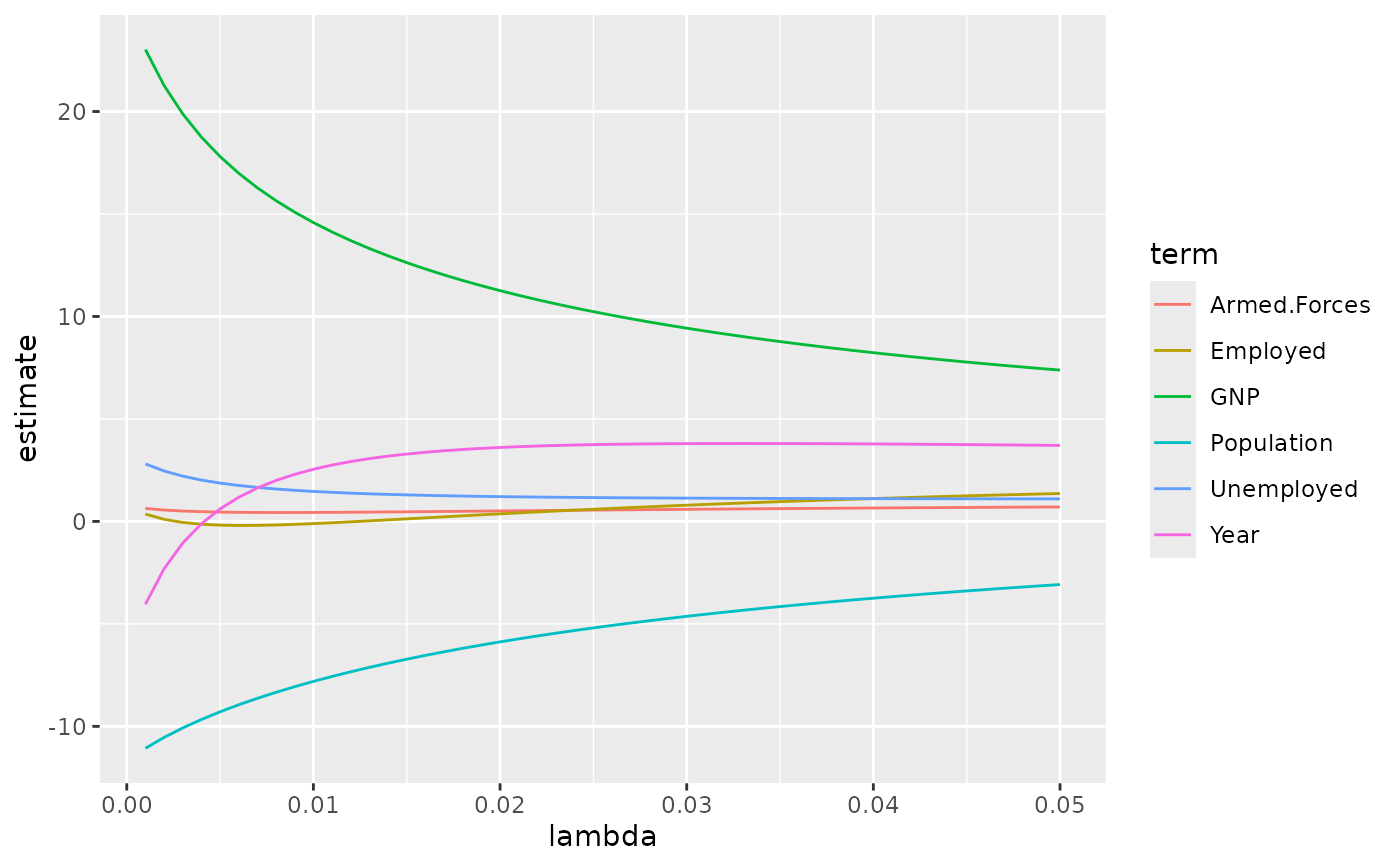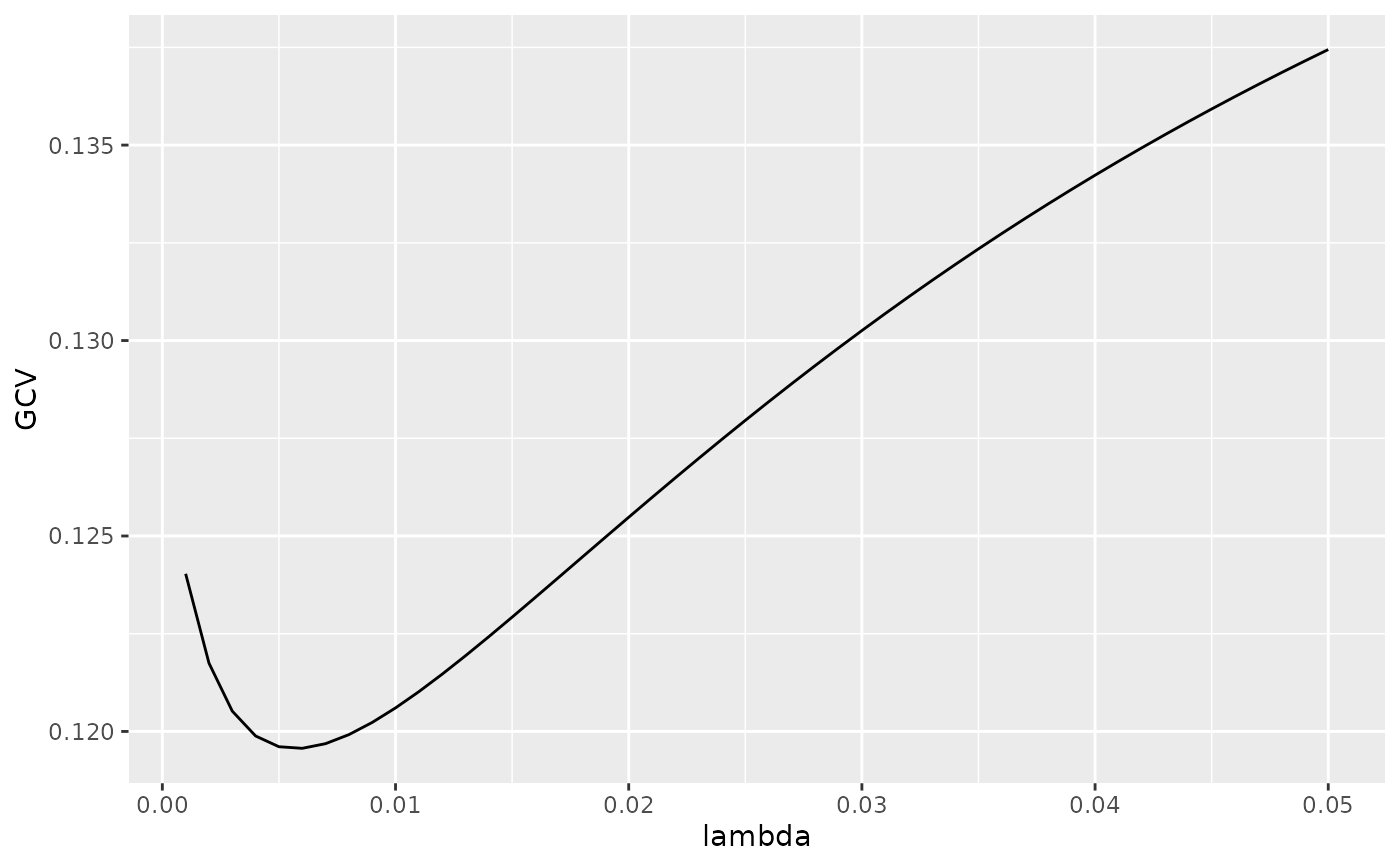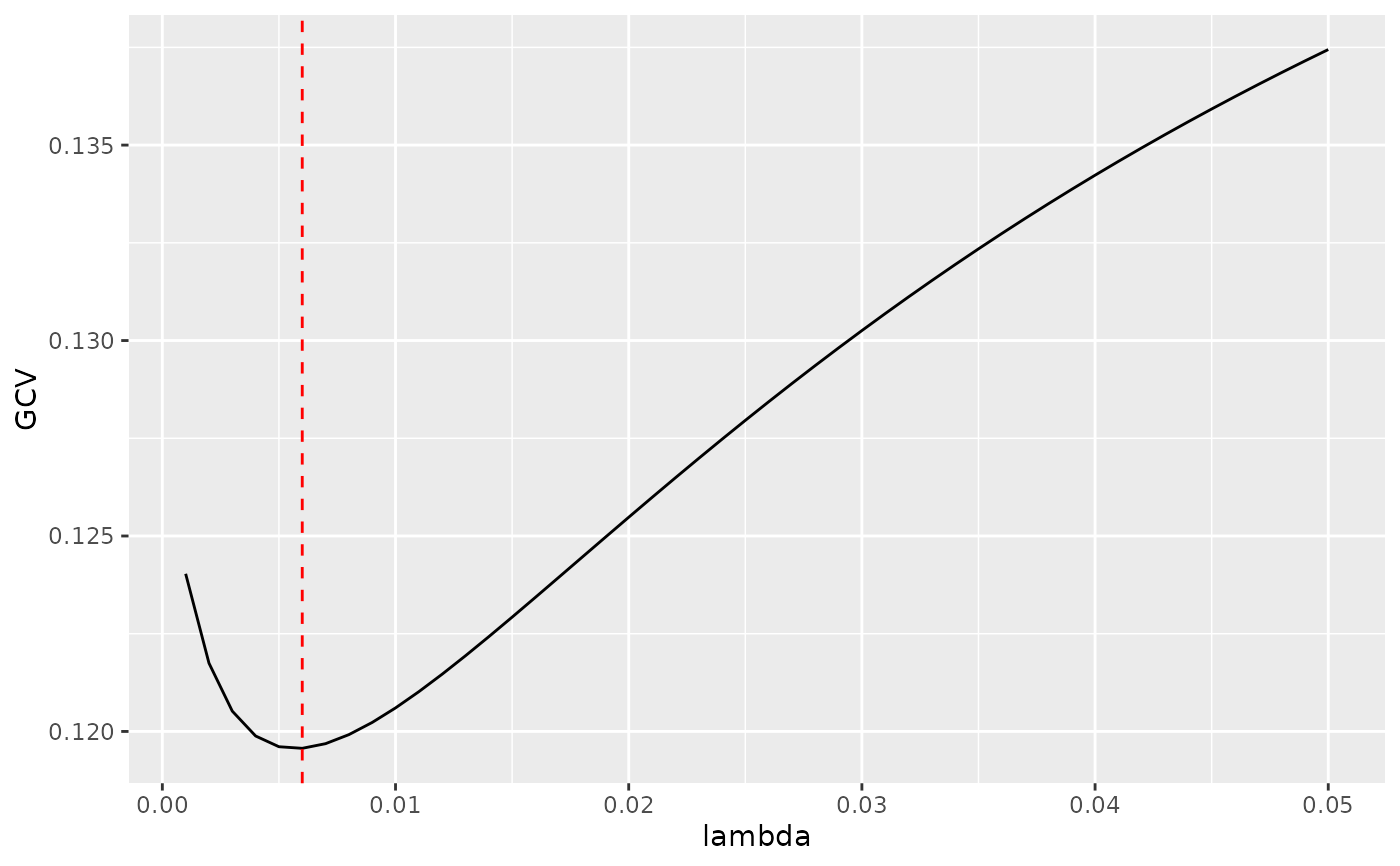Glance accepts a model object and returns a tibble::tibble() with exactly one row of model summaries. The summaries are typically goodness of fit measures, p-values for hypothesis tests on residuals, or model convergence information.

Glance never returns information from the original call to the modelling function. This includes the name of the modelling function or any arguments passed to the modelling function.

Glance does not calculate summary measures. Rather, it farms out these computations to appropriate methods and gathers the results together. Sometimes a goodness of fit measure will be undefined. In these cases the measure will be reported as NA.

# S3 method for ridgelm
glance(x, ...)

## Arguments

x A ridgelm object returned from MASS::lm.ridge(). Additional arguments. Not used. Needed to match generic signature only. Cautionary note: Misspelled arguments will be absorbed in ..., where they will be ignored. If the misspelled argument has a default value, the default value will be used. For example, if you pass conf.lvel = 0.9, all computation will proceed using conf.level = 0.95. Additionally, if you pass newdata = my_tibble to an augment() method that does not accept a newdata argument, it will use the default value for the data argument.

## Details

This is similar to the output of select.ridgelm, but it is returned rather than printed.

glance(), MASS::select.ridgelm(), MASS::lm.ridge()

Other ridgelm tidiers: tidy.ridgelm()

## Value

A tibble::tibble() with exactly one row and columns:

kHKB

modified HKB estimate of the ridge constant

kLW

modified L-W estimate of the ridge constant

lambdaGCV

choice of lambda that minimizes GCV

## Examples


names(longley) <- "y"
fit1 <- MASS::lm.ridge(y ~ ., longley)
tidy(fit1)#> # A tibble: 6 x 5
#>   lambda   GCV term         estimate scale
#>    <dbl> <dbl> <chr>           <dbl> <dbl>
#> 1      0 0.128 GNP            25.4   96.2
#> 2      0 0.128 Unemployed      3.30  90.5
#> 3      0 0.128 Armed.Forces    0.752 67.4
#> 4      0 0.128 Population    -11.7    6.74
#> 5      0 0.128 Year           -6.54   4.61
#> 6      0 0.128 Employed        0.786  3.40
fit2 <- MASS::lm.ridge(y ~ ., longley, lambda = seq(0.001, .05, .001))
td2 <- tidy(fit2)
g2 <- glance(fit2)

# coefficient plot
library(ggplot2)
ggplot(td2, aes(lambda, estimate, color = term)) +
geom_line()# GCV plot
ggplot(td2, aes(lambda, GCV)) +
geom_line()# add line for the GCV minimizing estimate
ggplot(td2, aes(lambda, GCV)) +
geom_line() +
geom_vline(xintercept = g2\$lambdaGCV, col = "red", lty = 2)